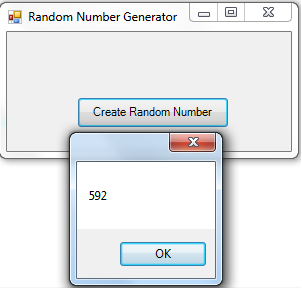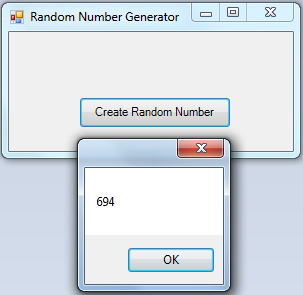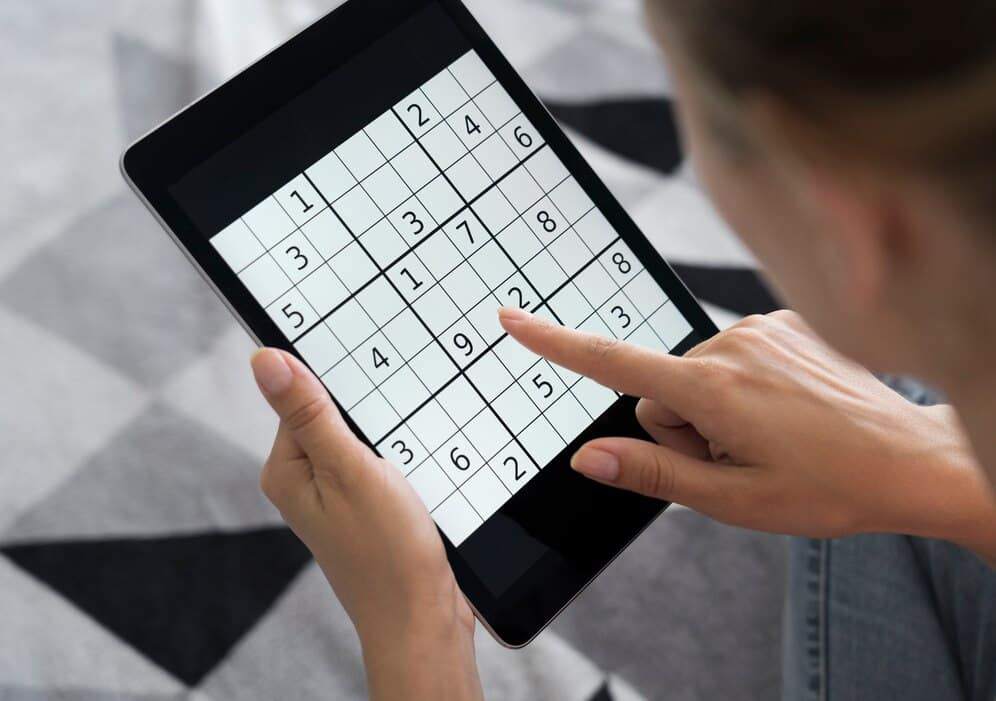Random Number

# Random Number Generator in C#

## Details: Random Number

Random numbers are numbers that appear to be unpredictable. In computer programming, random numbers are used to determine the outcome of calculations or the results of a game. For example, in a lottery, the results of a draw would be determined by the random number generator.

In this article, we will learn how to generate random numbers in the C# programming language and use them in our applications.

1 – We start by opening a Windows Form Application in Microsoft Visual Studio 2019 by following the directives;
– Navigate to File
– Click on New Project
– Select Windows Application, and,
– Save project as Random Number Generation

2 – We can now add the following;
– A button named Button1 and labelled as “Create Random Number”
Your inferface should look like the image below3 – Then, right in the code tab, generate a code for your Buttn1_Click. Type this code underneath:

```				```
public Random random = new Random();
public void Button1_Click(System.Object sender, System.EventArgs e)
{
int i = default(int);
for (i = 1; i <= 3; i++)
{
MessageBox.Show(Convert.ToString(random.Next(0, 1000)));
}
}
```
```

Let’s declare public Random random = new Random(); as the Global Variable for instantiating a Random Object new Random(); that holds the variable random. We also initiated Dim i As Integer as the integer so that  three random numbers (For i=1 to 3) can be generated when we tap the OK button in the MsgBox.

MessageBox.Show(Convert.ToString(random.Next(0, 1000))); the syntax is for displaying the random number. random.Next(0, 1000) initializes a random number from 0 to 1000, then the output is converted into a string using Convert.ToString syntax.

Once you click on the Button, the random number will be generated like on the image below;Again, press the OK button in the MessageBox. It will initiate another set of random numbers like this:Finally, tap the OK button. It will also create another set of random numbers like this:The program was made to create three numbers at a time such as i = 1-3

## Recent Posts## Complete School Management System using Python Django

July 8, 2022